# Testing the VI.ggplot() within the BrailleR package“

N.B. the commands here are either exact copies of the commands presented in Wickham (2009) or some minor alterations to them. Notably, some code given in the book no longer works. This is given a `#!`

The `ggplot2` package has a `summary` method that often but not always offers something to show that things have changed from one plot to another. Summary commands are included below but commented out.

``````library(BrailleR)
library(ggplot2)   ``````
``````##
## Attaching package: 'ggplot2'``````
``````## The following objects are masked from 'package:BrailleR':
##
##     xlab, ylab``````
``````library(magrittr)
dsmall = diamonds[1:100,]   ``````
``````g1 = qplot(carat, price, data = diamonds)
# summary(g1)
g1  ``````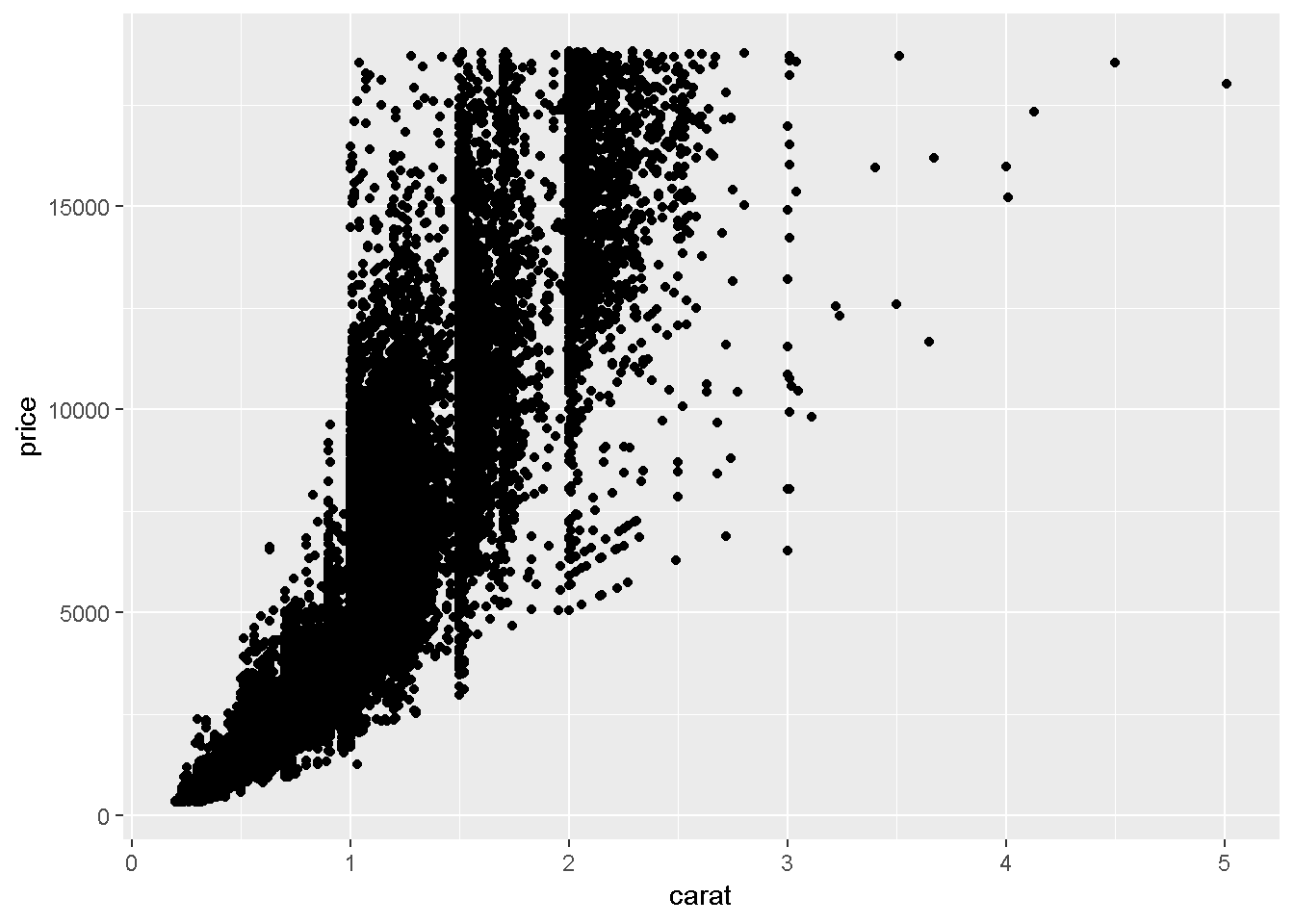``VI(g1)  ``
``````## This is an untitled chart with no subtitle or caption.
## It has x-axis 'carat' with labels 0, 1, 2, 3, 4 and 5.
## It has y-axis 'price' with labels 0, 5000, 10000 and 15000.
## The chart is a set of 53940 points.``````
``````g2 = qplot(carat, price, data = dsmall, colour = color)
# summary(g2)
g2``````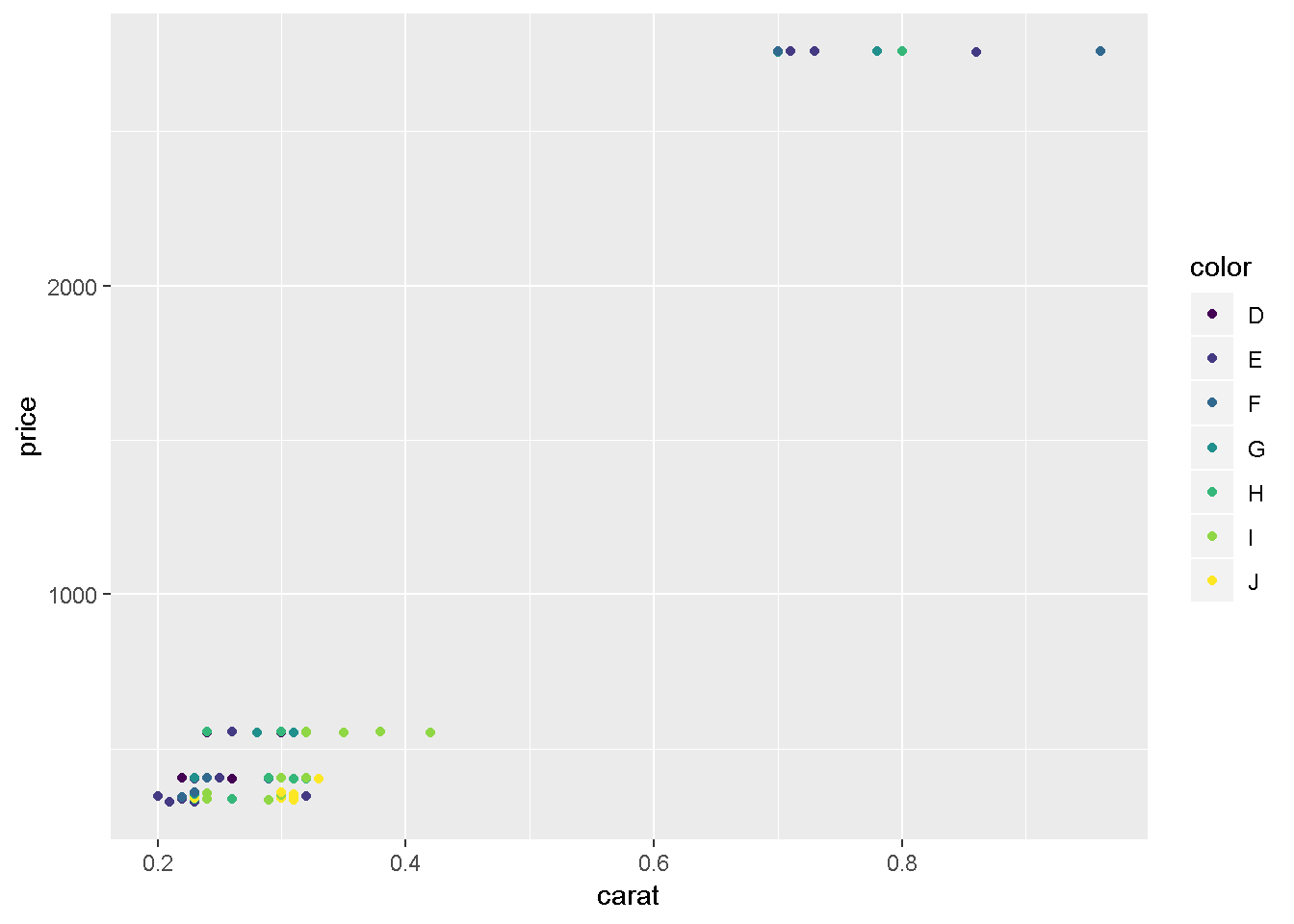``g2 %>% VI()   ``
``````## This is an untitled chart with no subtitle or caption.
## It has x-axis 'carat' with labels 0.2, 0.4, 0.6 and 0.8.
## It has y-axis 'price' with labels 1000 and 2000.
## There is a legend indicating that colour is used to represent color, with 7 levels:
## D represented by colour very deep purple,
## E represented by colour vivid purplish blue,
## F represented by colour moderate blue,
## G represented by colour vivid bluish green,
## H represented by colour brilliant green,
## I represented by colour vivid yellow green and
## J represented by colour vivid greenish yellow.
## The chart is a set of 100 points.``````
``````# to get the content of the graph to be printed we need:
#class(g2)="list"; g2   ``````
``````g3 = qplot(carat, price, data = dsmall, shape = cut)
# summary(g3)
g3``````
``## Warning: Using shapes for an ordinal variable is not advised``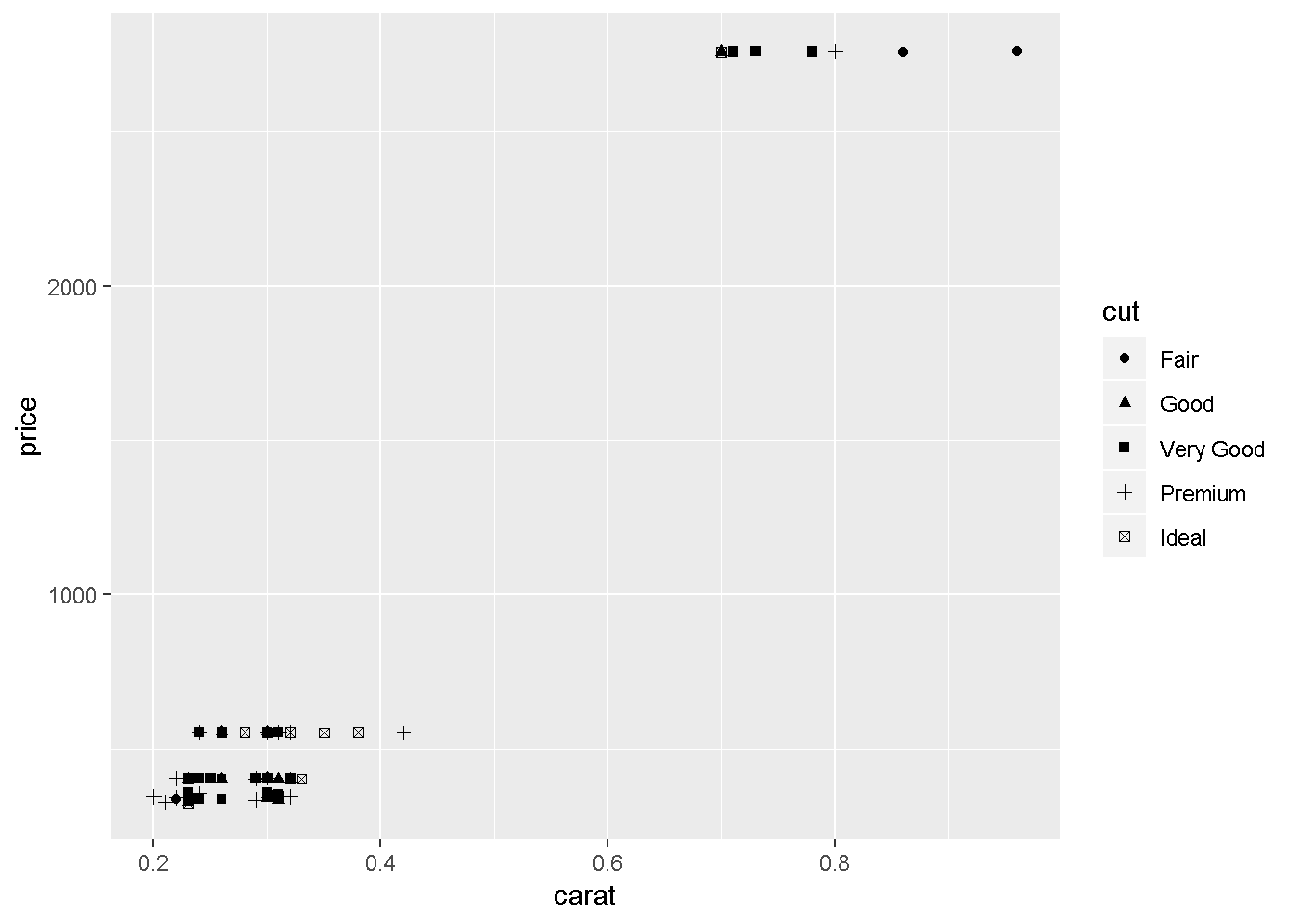``VI(g3)   ``
``## Warning: Using shapes for an ordinal variable is not advised``
``````## This is an untitled chart with no subtitle or caption.
## It has x-axis 'carat' with labels 0.2, 0.4, 0.6 and 0.8.
## It has y-axis 'price' with labels 1000 and 2000.
## There is a legend indicating that shape is used to represent cut, with 5 levels:
## Fair represented by shape solid circle,
## Good represented by shape solid triangle,
## Very Good represented by shape solid square,
## Premium represented by shape plus and
## Ideal represented by shape boxed X.
## The chart is a set of 100 points.``````
``#class(g3)="list"; g3   ``
``````# to get semi-transparent points
g4 = qplot(carat, price, data = diamonds, alpha = I(1/100))
# summary(g4)
g4``````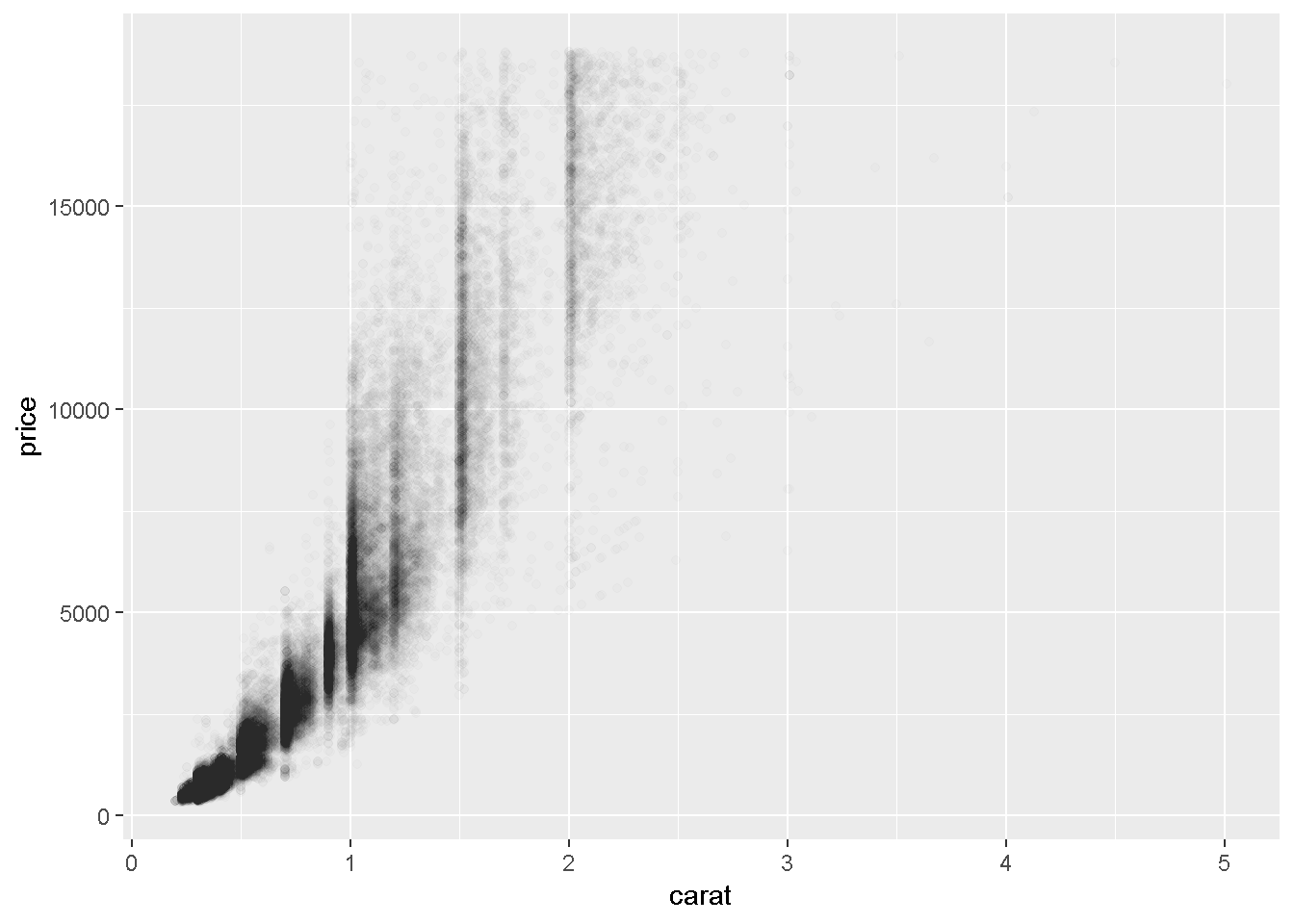``VI(g4)   ``
``````## This is an untitled chart with no subtitle or caption.
## It has x-axis 'carat' with labels 0, 1, 2, 3, 4 and 5.
## It has y-axis 'price' with labels 0, 5000, 10000 and 15000.
## The chart is a set of 53940 points.
## The chart has alpha set to 0.01.``````
``````# to add a smoother (default is loess for n<1000)
g5 = qplot(carat, price, data = dsmall, geom = c("point", "smooth"))
# summary(g5)
g5``````
``## `geom_smooth()` using method = 'loess' and formula 'y ~ x'``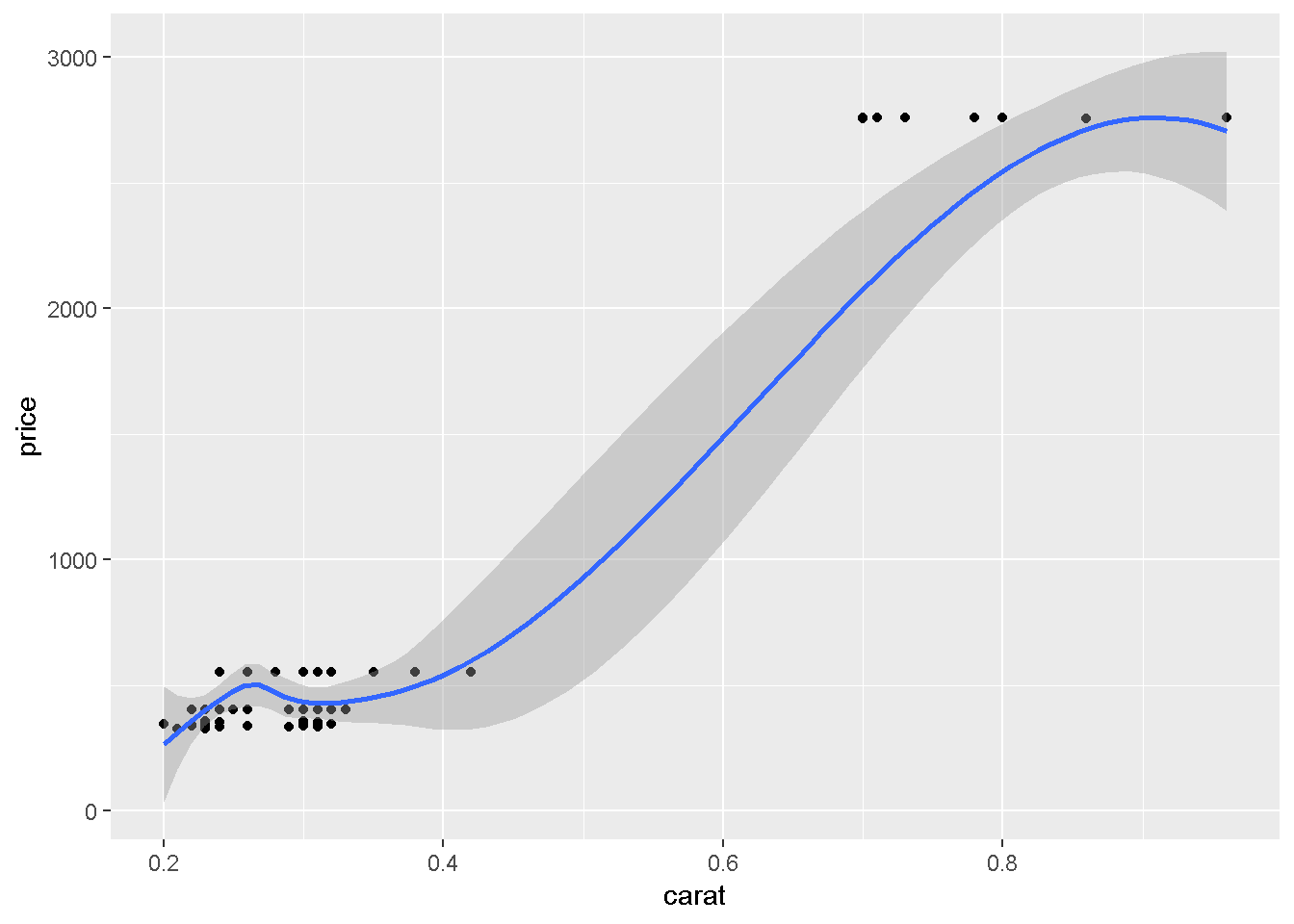``VI(g5)   ``
``````## This is an untitled chart with no subtitle or caption.
## It has x-axis 'carat' with labels 0.2, 0.4, 0.6 and 0.8.
## It has y-axis 'price' with labels 0, 1000, 2000 and 3000.
## It has 2 layers.
## Layer 1 is a set of 100 points.
## Layer 2 is a smoothed curve using method 'auto' with confidence intervals.``````
``````#! g5a = qplot(carat, price, data = dsmall, geom = c("point", "smooth"), span = 1)
library(splines)
#! g5b = qplot(carat, price, data = dsmall, geom = c("point", "smooth"), method = "lm")
#! g5c = qplot(carat, price, data = dsmall, geom = c("point", "smooth"), method = "lm", formula = y ~ ns(x,5))    ``````
``````# continuous v categorical
g6 = qplot(color, price / carat, data = diamonds, geom = "jitter", alpha = I(1 / 50))
# summary(g6)
g6``````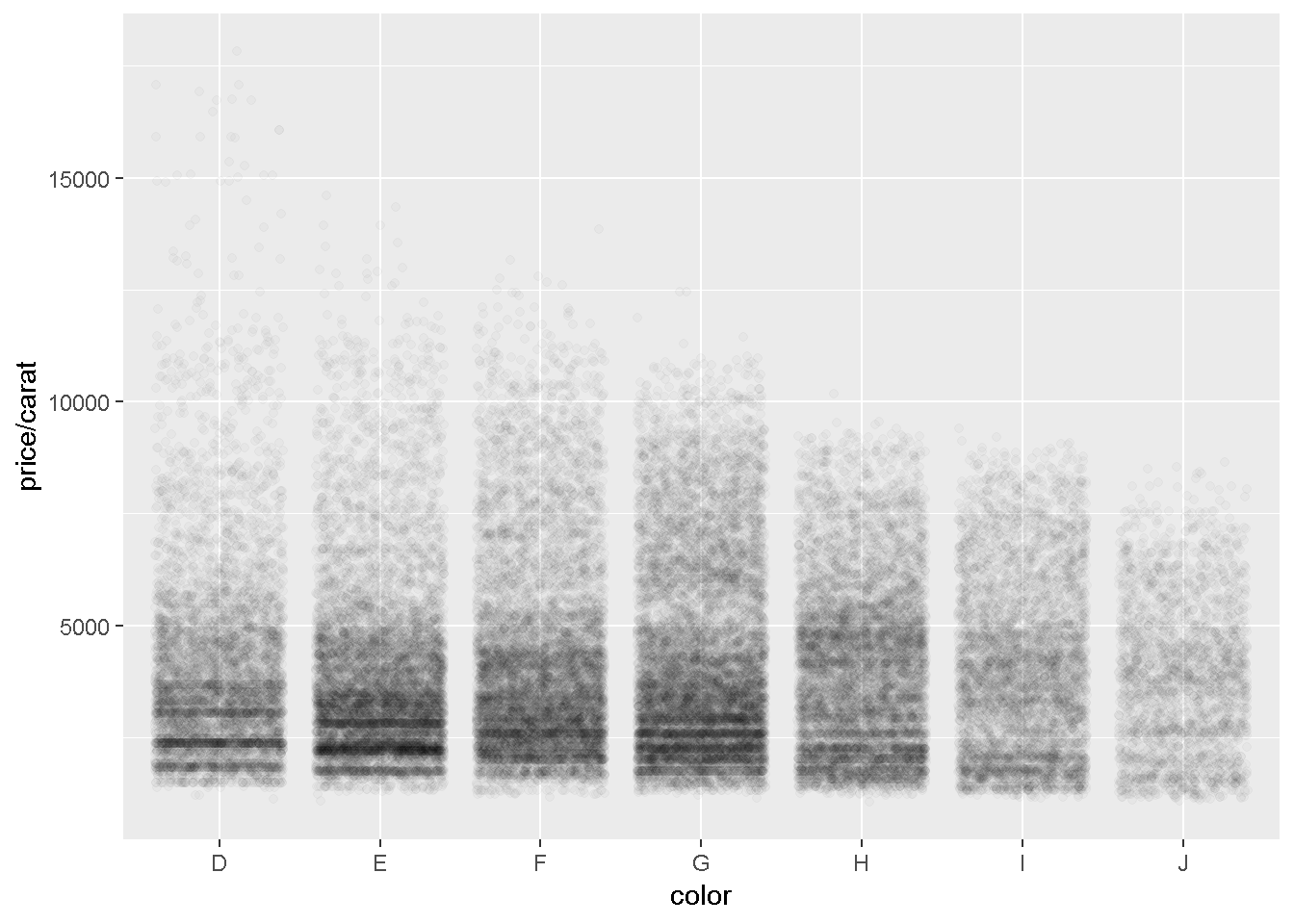``VI(g6)   ``
``````## This is an untitled chart with no subtitle or caption.
## It has x-axis 'color' with labels D, E, F, G, H, I and J.
## It has y-axis 'price/carat' with labels 5000, 10000 and 15000.
## The chart is a set of 53940 points.
## The chart has alpha set to 0.02.``````
``````g6a = qplot(color, price / carat, data = diamonds, geom = "boxplot")
# summary(g6a)
g6a``````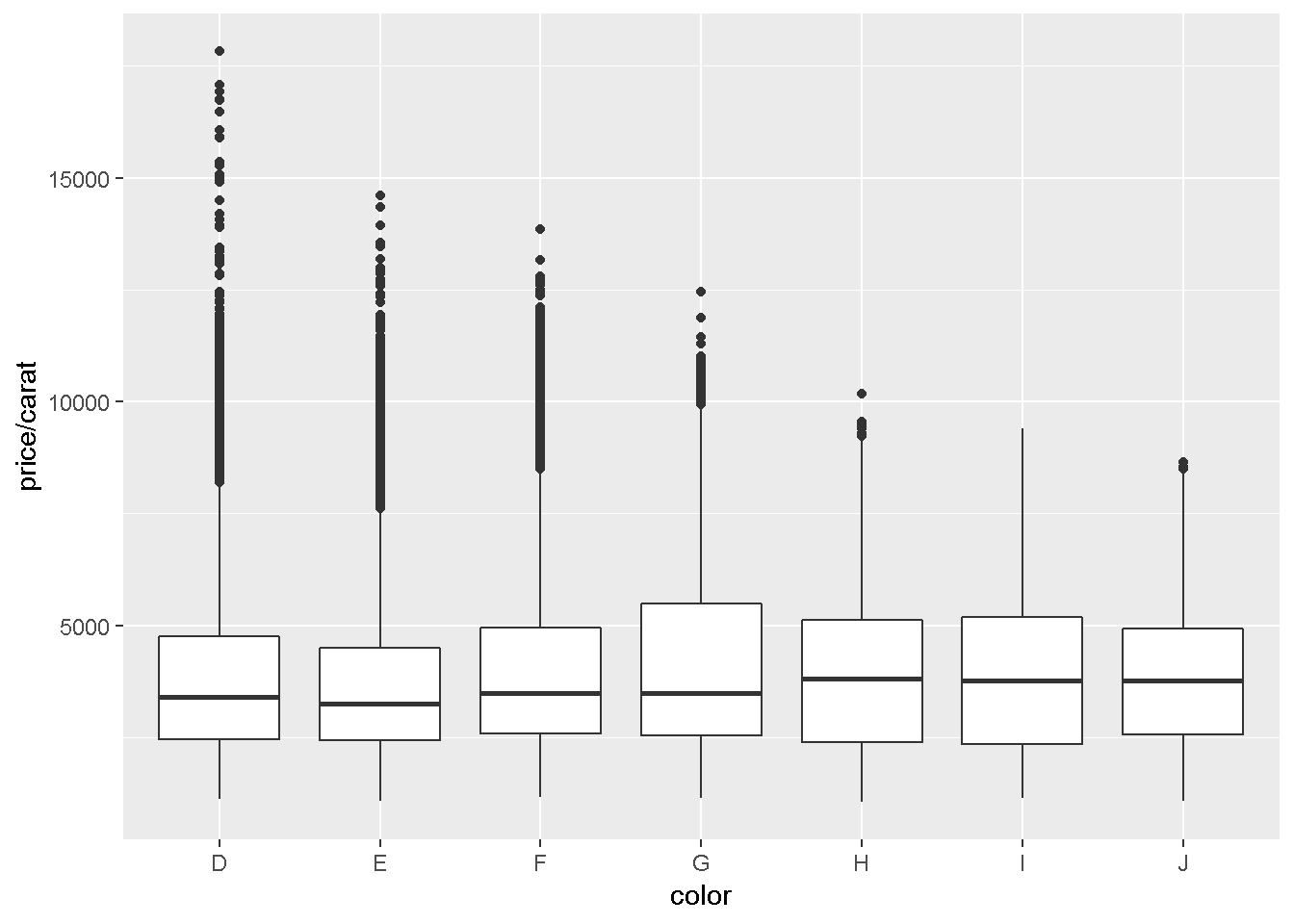``VI(g6a)   ``
``````## This is an untitled chart with no subtitle or caption.
## It has x-axis 'color' with labels D, E, F, G, H, I and J.
## It has y-axis 'price/carat' with labels 5000, 10000 and 15000.
## The chart is a boxplot comprised of 7 boxes with whiskers.
## There is a box at x=D.
## It has median 3410.53. The box goes from 2455 to 4749.31, and the whiskers extend to 1128.12 and 8183.33.
## There are 338 outliers for this boxplot.
## There is a box at x=E.
## It has median 3253.66. The box goes from 2430.3 to 4508.41, and the whiskers extend to 1078.12 and 7616.39.
## There are 593 outliers for this boxplot.
## There is a box at x=F.
## It has median 3494.32. The box goes from 2587.1 to 4947.22, and the whiskers extend to 1168 and 8477.5.
## There are 585 outliers for this boxplot.
## There is a box at x=G.
## It has median 3490.38. The box goes from 2538.24 to 5500, and the whiskers extend to 1139.02 and 9937.2.
## There are 119 outliers for this boxplot.
## There is a box at x=H.
## It has median 3818.89. The box goes from 2396.88 to 5127.28, and the whiskers extend to 1051.16 and 9220.
## There are 13 outliers for this boxplot.
## There is a box at x=I.
## It has median 3779.74. The box goes from 2344.65 to 5196.75, and the whiskers extend to 1151.72 and 9397.5.
## There are 0 outliers for this boxplot.
## There is a box at x=J.
## It has median 3780. The box goes from 2562.87 to 4927.95, and the whiskers extend to 1080.65 and 8426.13.
## There are 3 outliers for this boxplot.``````
``````# univariate plots
g7a = qplot(carat, data = diamonds, geom = "histogram")
# summary(g7a)
g7a``````
``## `stat_bin()` using `bins = 30`. Pick better value with `binwidth`.``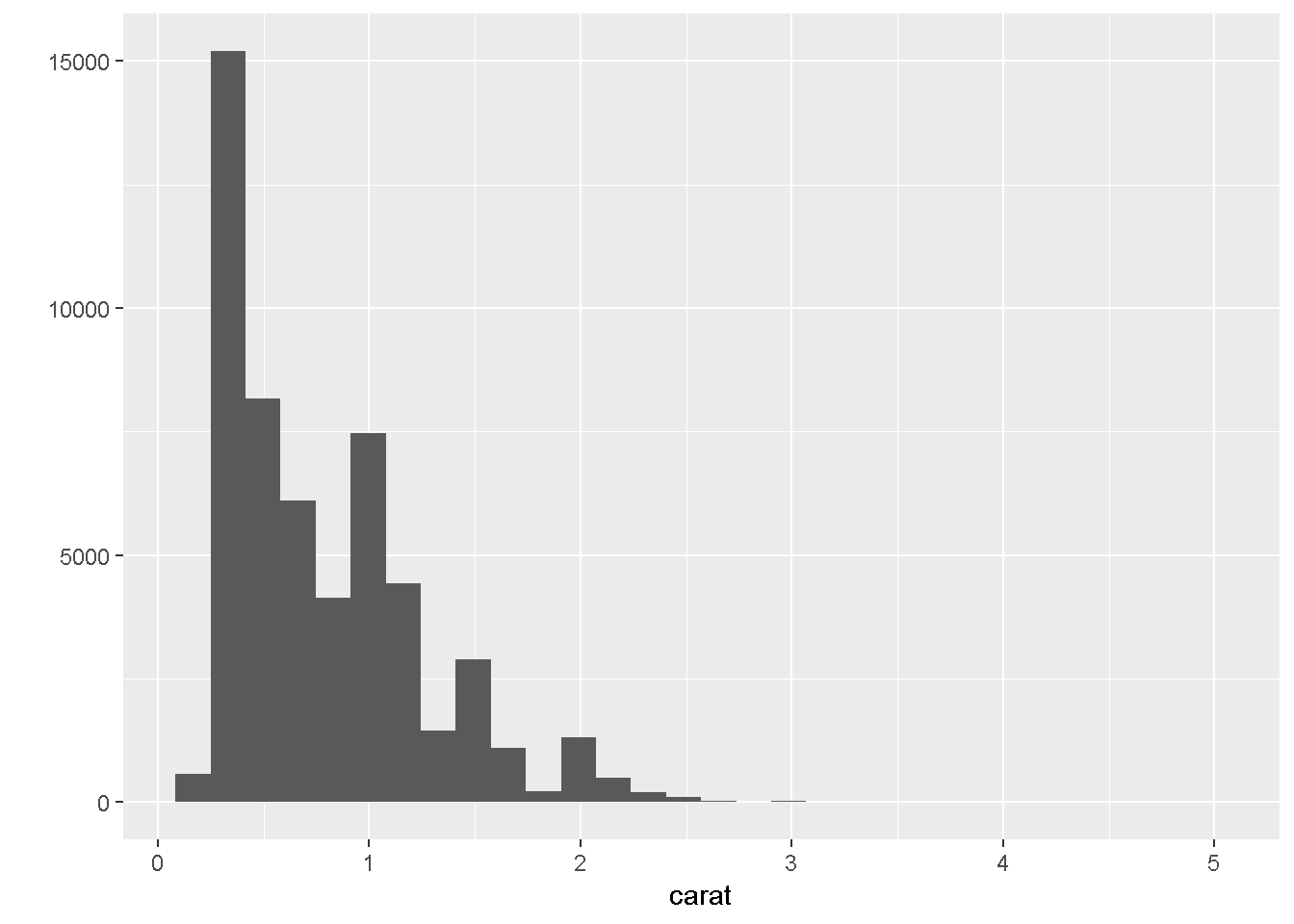``VI(g7a)   ``
``````## This is an untitled chart with no subtitle or caption.
## It has x-axis 'carat' with labels 0, 1, 2, 3, 4 and 5.
## It has y-axis '' with labels 0, 5000, 10000 and 15000.
## The chart is a bar chart containing 30 vertical bars.``````
``````g7b = qplot(carat, data = diamonds, geom = "histogram", binwidth = 1, xlim = c(0,3))
g7b``````
``## Warning: Removed 32 rows containing non-finite values (stat_bin).``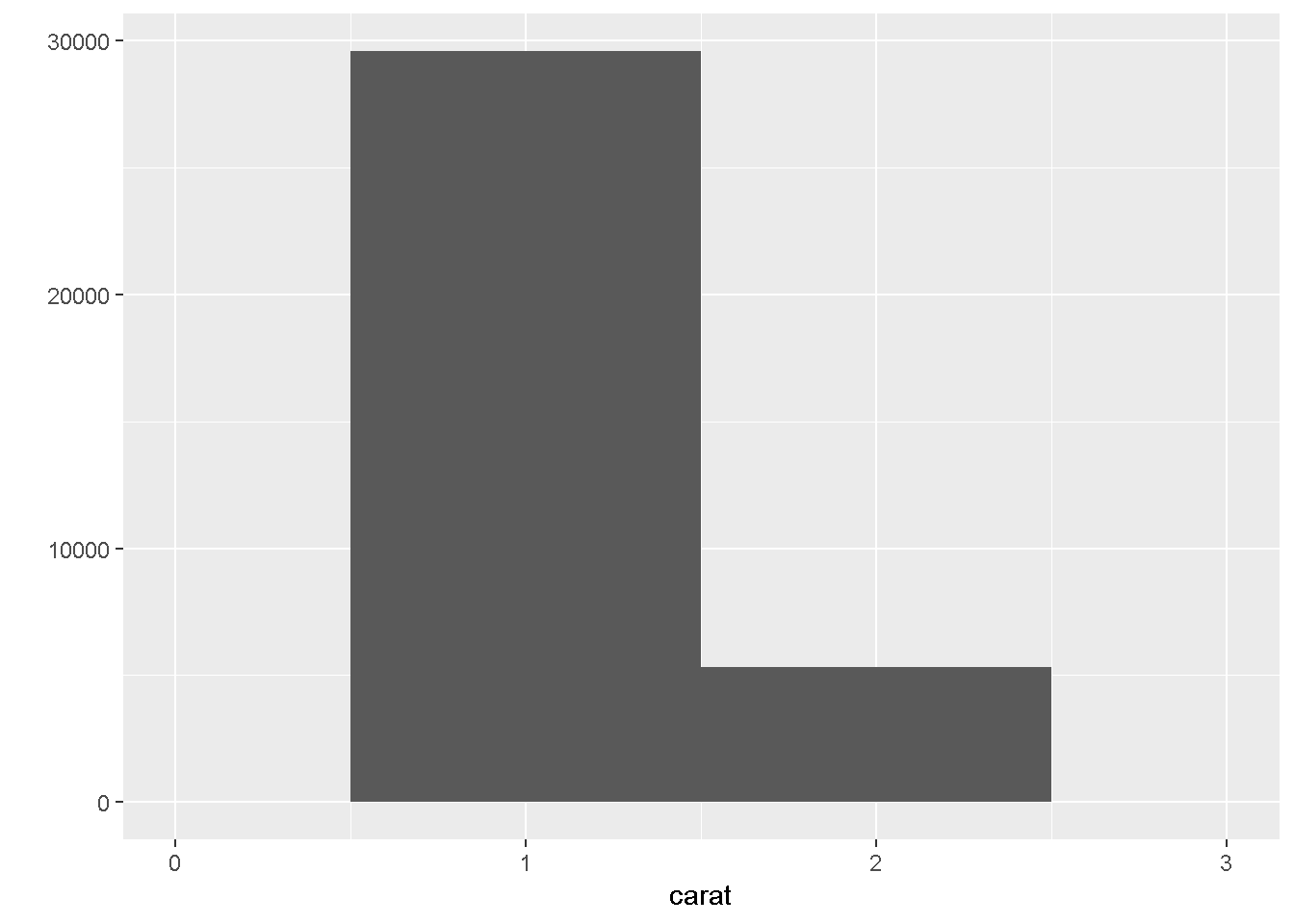``VI(g7b)   ``
``## Warning: Removed 32 rows containing non-finite values (stat_bin).``
``````## This is an untitled chart with no subtitle or caption.
## It has x-axis 'carat' with labels 0, 1, 2 and 3.
## It has y-axis '' with labels 0, 10000, 20000 and 30000.
## The chart is a bar chart containing 2 vertical bars.
## Bar 1 is centered horizontally at 1, and spans vertically from 0 to 29566.
## Bar 2 is centered horizontally at 2, and spans vertically from 0 to 5316.``````
``````g7c = qplot(carat, data = diamonds, geom = "histogram", binwidth = 0.1, xlim = c(0,3))
g7c``````
``## Warning: Removed 32 rows containing non-finite values (stat_bin).``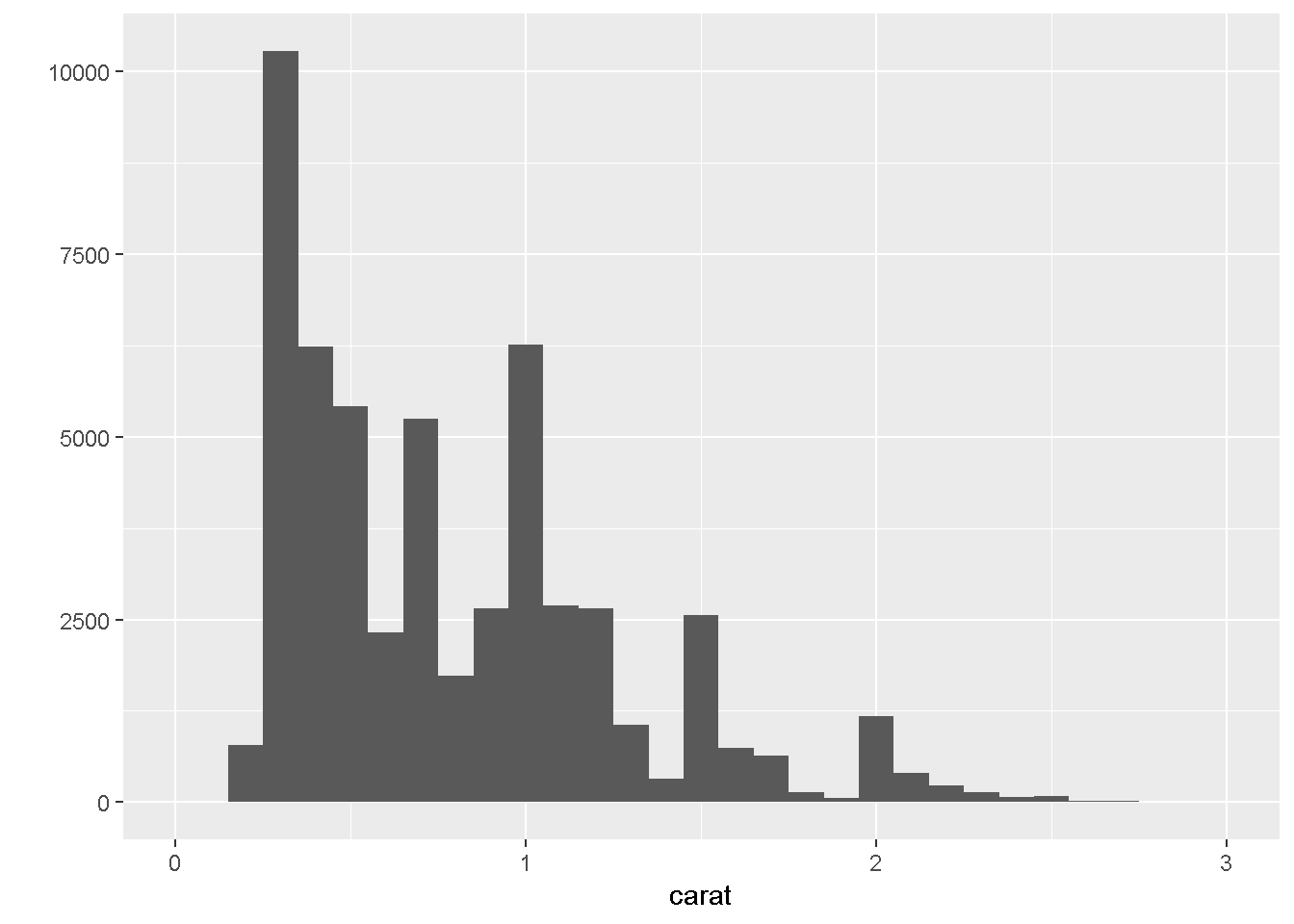``VI(g7c)   ``
``## Warning: Removed 32 rows containing non-finite values (stat_bin).``
``````## This is an untitled chart with no subtitle or caption.
## It has x-axis 'carat' with labels 0, 1, 2 and 3.
## It has y-axis '' with labels 0, 2500, 5000, 7500 and 10000.
## The chart is a bar chart containing 29 vertical bars.``````
``````g7d = qplot(carat, data = diamonds, geom = "histogram", binwidth = 0.01, xlim = c(0,3))
# summary(g7d)
g7d``````
``## Warning: Removed 32 rows containing non-finite values (stat_bin).``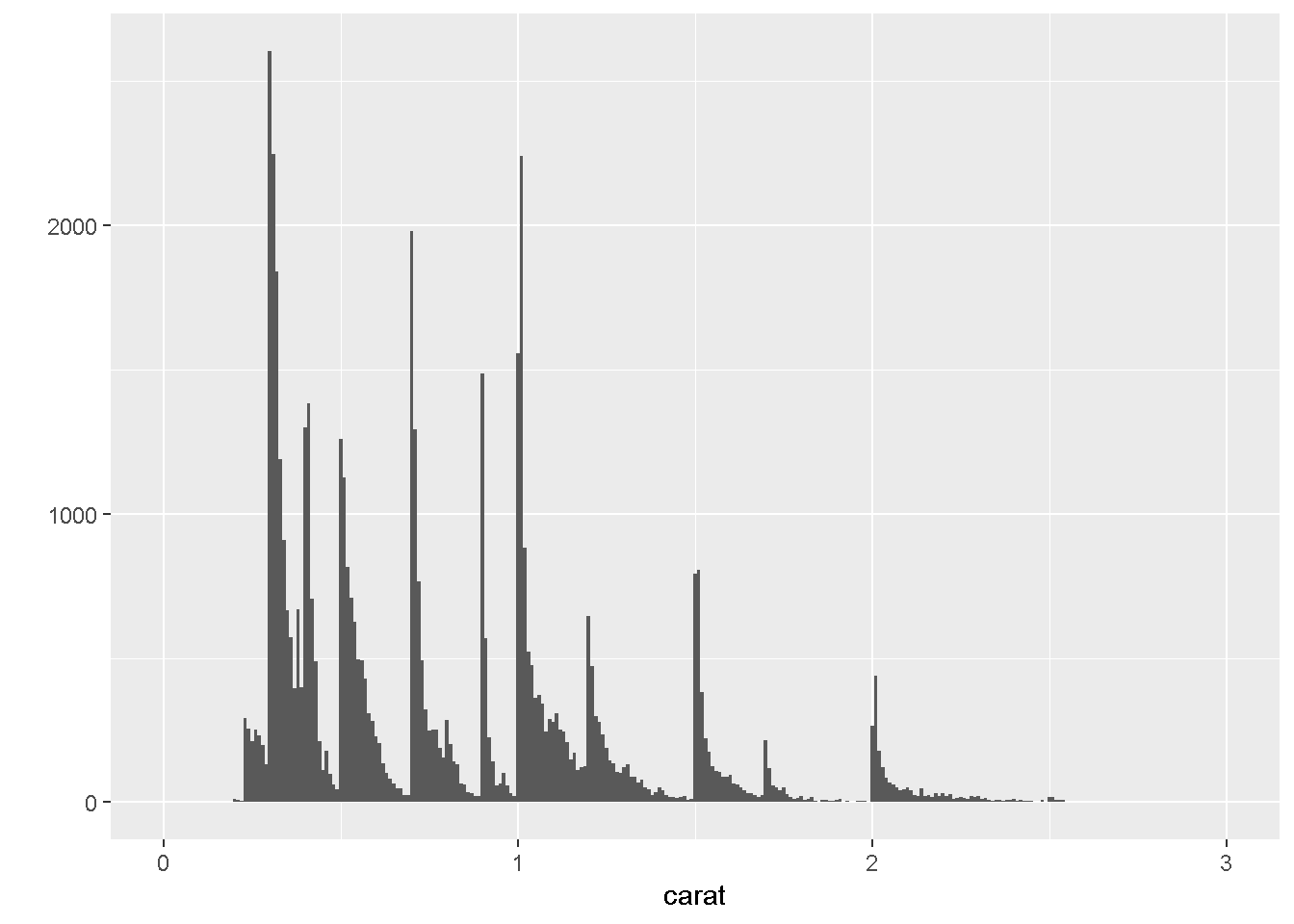``VI(g7d)   ``
``## Warning: Removed 32 rows containing non-finite values (stat_bin).``
``````## This is an untitled chart with no subtitle or caption.
## It has x-axis 'carat' with labels 0, 1, 2 and 3.
## It has y-axis '' with labels 0, 1000 and 2000.
## The chart is a bar chart containing 299 vertical bars.``````
``````g8 = qplot(carat, data = diamonds, geom = "density")
# summary(g8)
g8``````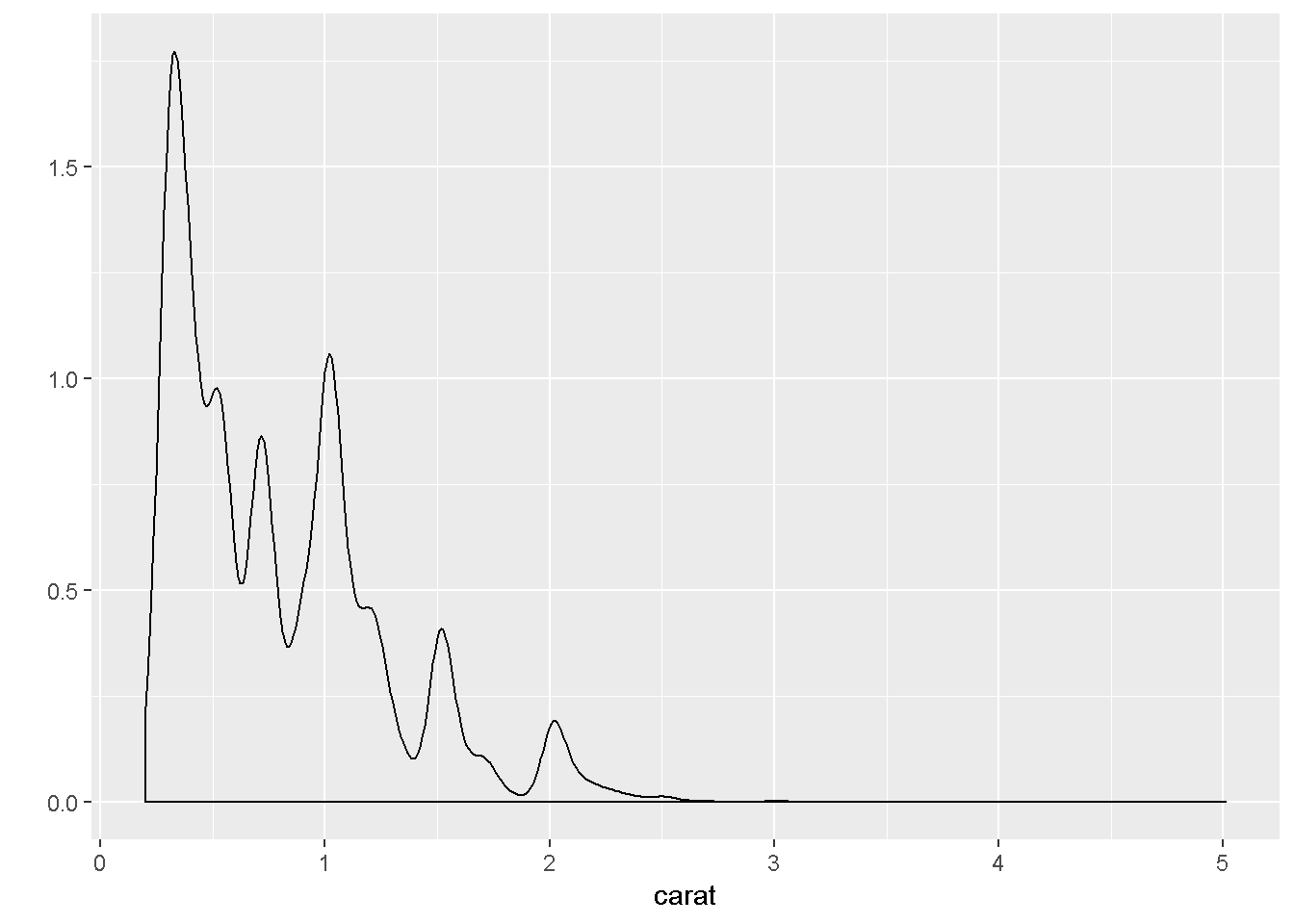``VI(g8)   ``
``````## This is an untitled chart with no subtitle or caption.
## It has x-axis 'carat' with labels 0, 1, 2, 3, 4 and 5.
## It has y-axis '' with labels 0.0, 0.5, 1.0 and 1.5.
## The chart is a type that VI isn't able to process.``````
``````# data is separated by implication using the following...
g9 = qplot(carat, data = diamonds, geom = "density", colour = color)
# summary(g9)
g9``````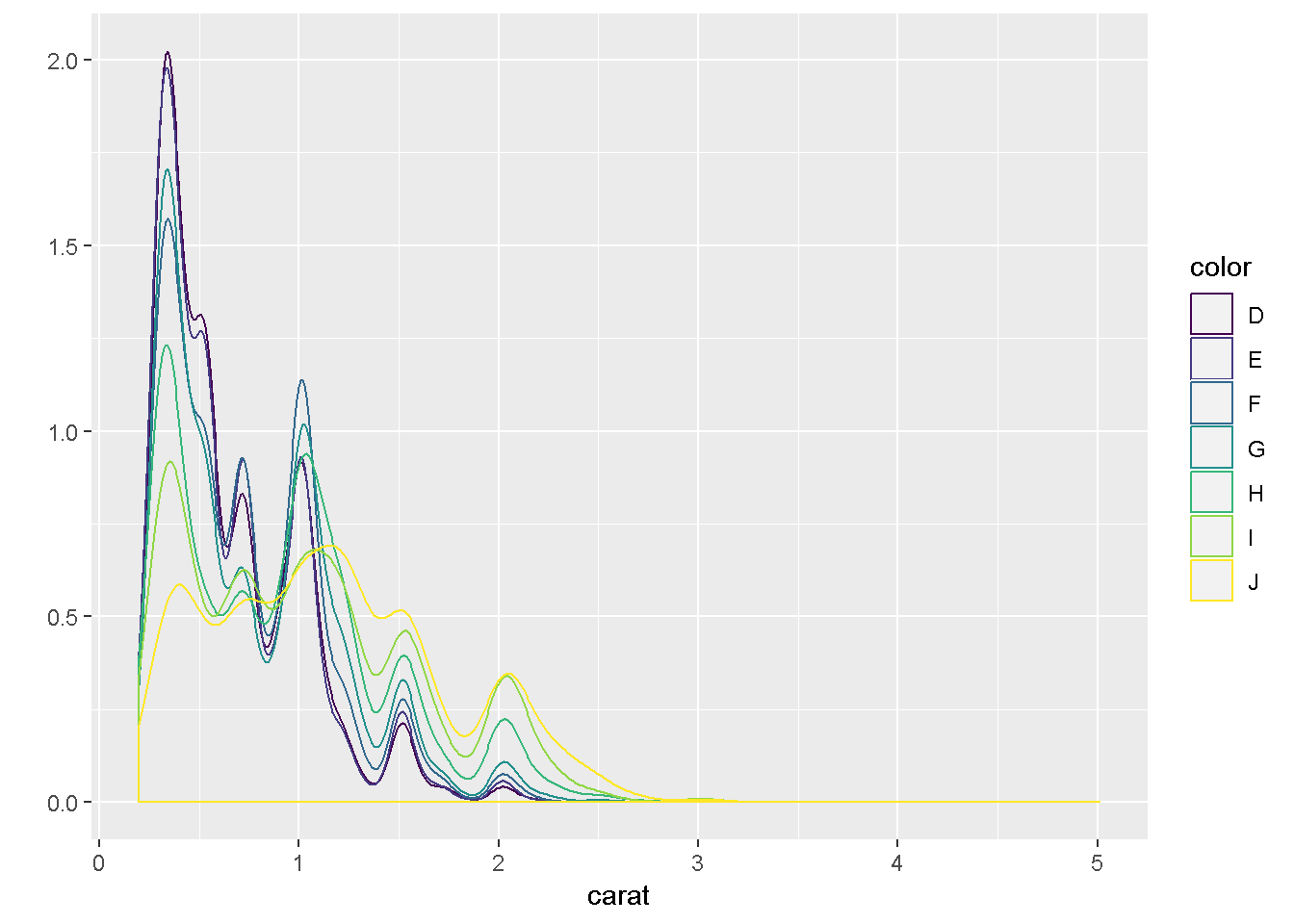``VI(g9)   ``
``````## This is an untitled chart with no subtitle or caption.
## It has x-axis 'carat' with labels 0, 1, 2, 3, 4 and 5.
## It has y-axis '' with labels 0.0, 0.5, 1.0, 1.5 and 2.0.
## There is a legend indicating that colour is used to represent color, with 7 levels:
## D represented by colour very deep purple,
## E represented by colour vivid purplish blue,
## F represented by colour moderate blue,
## G represented by colour vivid bluish green,
## H represented by colour brilliant green,
## I represented by colour vivid yellow green and
## J represented by colour vivid greenish yellow.
## The chart is a type that VI isn't able to process.``````
``````g10 = qplot(carat, data = diamonds, geom = "histogram", fill = color)
# summary(g10)
g10``````
``## `stat_bin()` using `bins = 30`. Pick better value with `binwidth`.``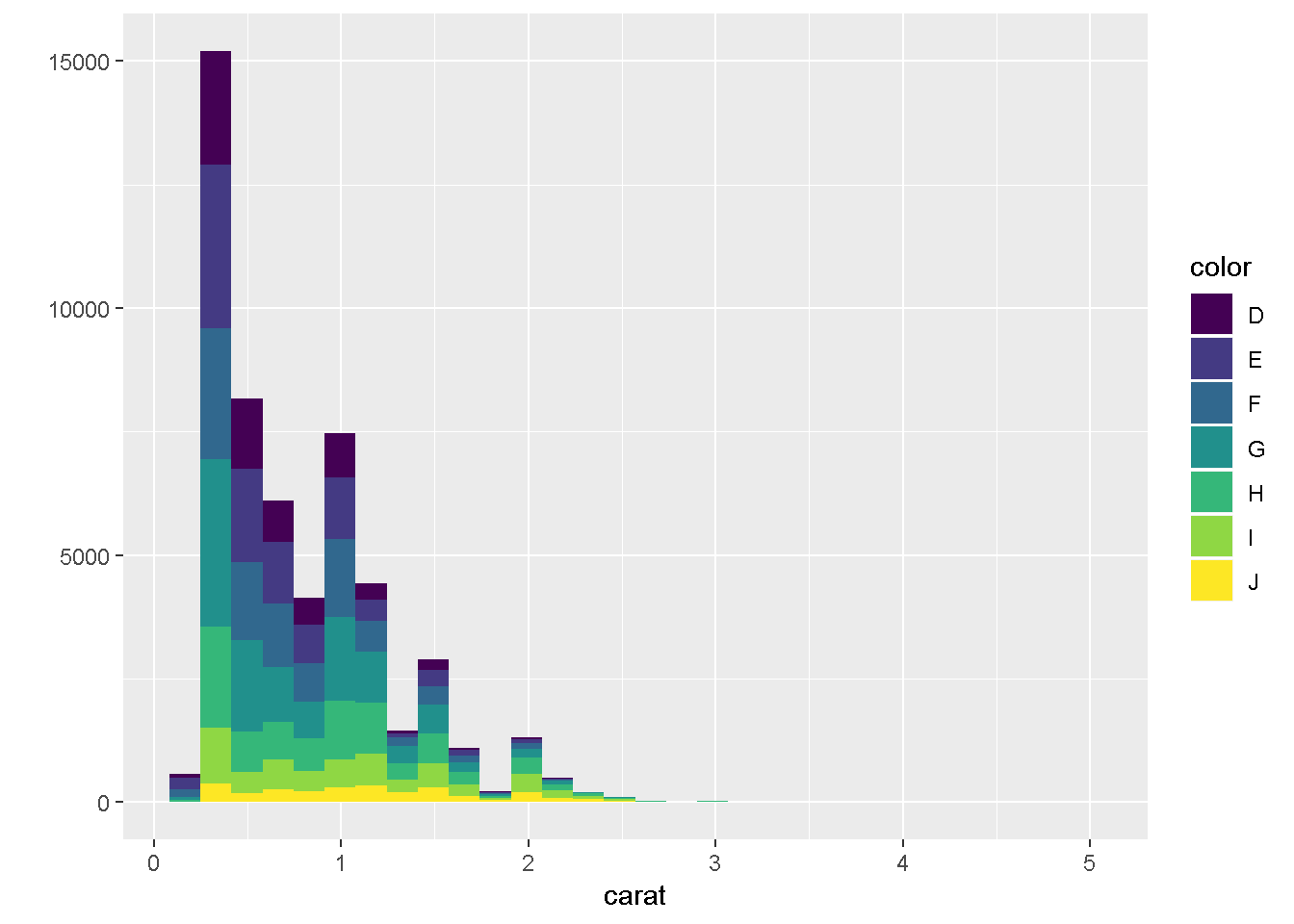``VI(g10)   ``
``````## This is an untitled chart with no subtitle or caption.
## It has x-axis 'carat' with labels 0, 1, 2, 3, 4 and 5.
## It has y-axis '' with labels 0, 5000, 10000 and 15000.
## There is a legend indicating that fill is used to represent color, with 7 levels:
## D represented by fill very deep purple,
## E represented by fill vivid purplish blue,
## F represented by fill moderate blue,
## G represented by fill vivid bluish green,
## H represented by fill brilliant green,
## I represented by fill vivid yellow green and
## J represented by fill vivid greenish yellow.
## The chart is a bar chart containing 210 vertical bars.``````
``````# bar charts for categorical variable
g11a = qplot(color, data = diamonds)
# summary(g11a)
g11a``````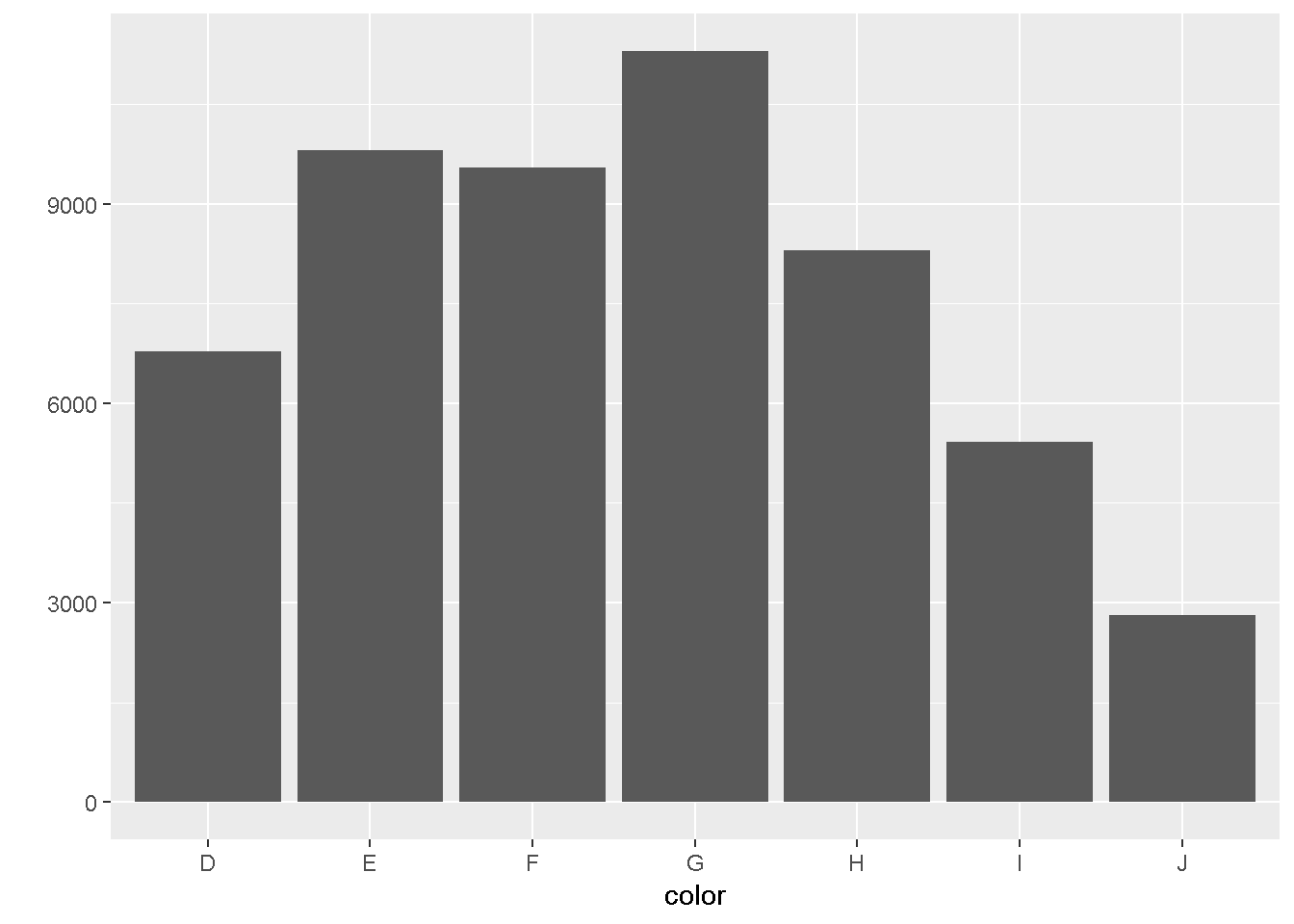``VI(g11a)   ``
``````## This is an untitled chart with no subtitle or caption.
## It has x-axis 'color' with labels D, E, F, G, H, I and J.
## It has y-axis '' with labels 0, 3000, 6000 and 9000.
## The chart is a bar chart containing 7 vertical bars.
## Bar 1 is centered horizontally at D, and spans vertically from 0 to 6775.
## Bar 2 is centered horizontally at E, and spans vertically from 0 to 9797.
## Bar 3 is centered horizontally at F, and spans vertically from 0 to 9542.
## Bar 4 is centered horizontally at G, and spans vertically from 0 to 11292.
## Bar 5 is centered horizontally at H, and spans vertically from 0 to 8304.
## Bar 6 is centered horizontally at I, and spans vertically from 0 to 5422.
## Bar 7 is centered horizontally at J, and spans vertically from 0 to 2808.``````
``````g11b = qplot(color, data = diamonds, geom = "bar")
# summary(g11b)
g11b````````VI(g11b)   ``
``````## This is an untitled chart with no subtitle or caption.
## It has x-axis 'color' with labels D, E, F, G, H, I and J.
## It has y-axis '' with labels 0, 3000, 6000 and 9000.
## The chart is a bar chart containing 7 vertical bars.
## Bar 1 is centered horizontally at D, and spans vertically from 0 to 6775.
## Bar 2 is centered horizontally at E, and spans vertically from 0 to 9797.
## Bar 3 is centered horizontally at F, and spans vertically from 0 to 9542.
## Bar 4 is centered horizontally at G, and spans vertically from 0 to 11292.
## Bar 5 is centered horizontally at H, and spans vertically from 0 to 8304.
## Bar 6 is centered horizontally at I, and spans vertically from 0 to 5422.
## Bar 7 is centered horizontally at J, and spans vertically from 0 to 2808.``````
``````g12a = qplot(color, data = diamonds, geom = "bar", weight = carat)
# summary(g12a)
g12a``````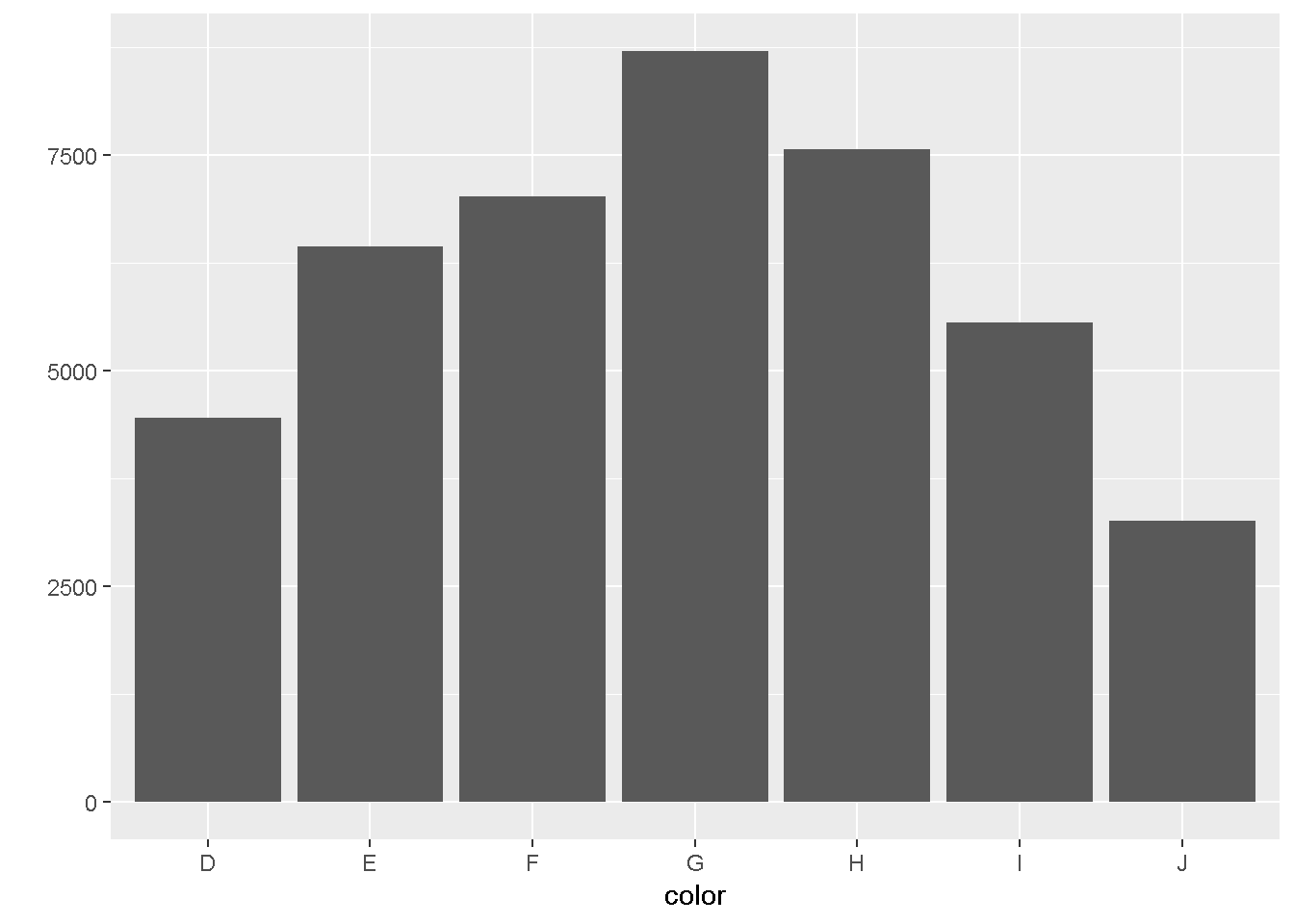``VI(g12a)   ``
``````## This is an untitled chart with no subtitle or caption.
## It has x-axis 'color' with labels D, E, F, G, H, I and J.
## It has y-axis '' with labels 0, 2500, 5000 and 7500.
## The chart is a bar chart containing 7 vertical bars.
## Bar 1 is centered horizontally at D, and spans vertically from 0 to 4456.56.
## Bar 2 is centered horizontally at E, and spans vertically from 0 to 6445.12.
## Bar 3 is centered horizontally at F, and spans vertically from 0 to 7028.05.
## Bar 4 is centered horizontally at G, and spans vertically from 0 to 8708.28.
## Bar 5 is centered horizontally at H, and spans vertically from 0 to 7571.58.
## Bar 6 is centered horizontally at I, and spans vertically from 0 to 5568.
## Bar 7 is centered horizontally at J, and spans vertically from 0 to 3263.28.``````
``````g12b = qplot(color, data = diamonds, geom = "bar", weight = carat) + scale_y_continuous("carat")
# summary(g12b)
g12b``````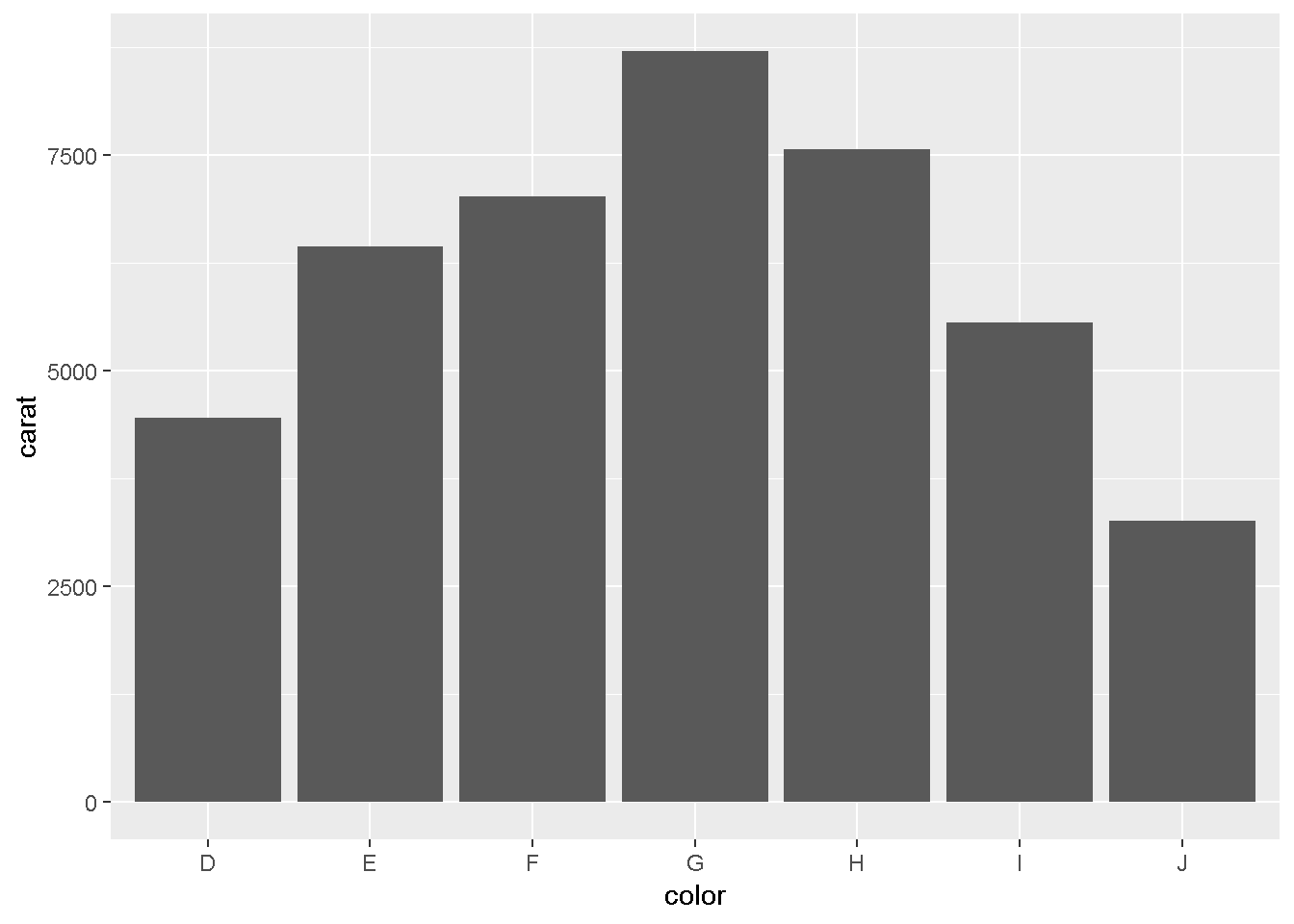``VI(g12b)   ``
``````## This is an untitled chart with no subtitle or caption.
## It has x-axis 'color' with labels D, E, F, G, H, I and J.
## It has y-axis '' with labels 0, 2500, 5000 and 7500.
## The chart is a bar chart containing 7 vertical bars.
## Bar 1 is centered horizontally at D, and spans vertically from 0 to 4456.56.
## Bar 2 is centered horizontally at E, and spans vertically from 0 to 6445.12.
## Bar 3 is centered horizontally at F, and spans vertically from 0 to 7028.05.
## Bar 4 is centered horizontally at G, and spans vertically from 0 to 8708.28.
## Bar 5 is centered horizontally at H, and spans vertically from 0 to 7571.58.
## Bar 6 is centered horizontally at I, and spans vertically from 0 to 5568.
## Bar 7 is centered horizontally at J, and spans vertically from 0 to 3263.28.``````
``````# time series plots
g13a = qplot(date, unemploy / pop, data = economics, geom = "line")
# summary(g13a)
g13a``````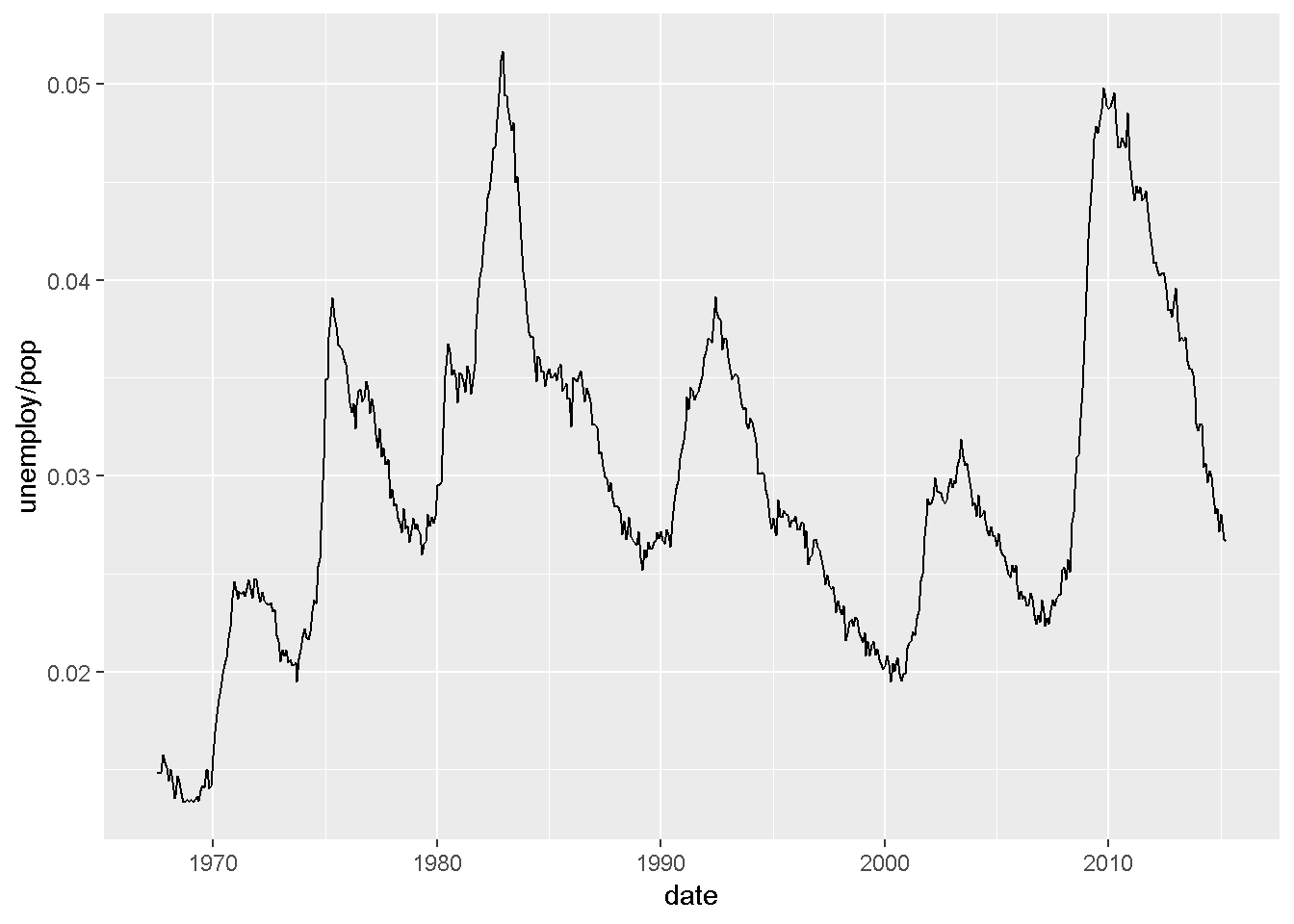``VI(g13a)   ``
``````## This is an untitled chart with no subtitle or caption.
## It has x-axis 'date' with labels 1970, 1980, 1990, 2000 and 2010.
## It has y-axis 'unemploy/pop' with labels 0.02, 0.03, 0.04 and 0.05.
## The chart is a set of 1 line.
## Line 1 connects 574 points.``````
``````g13b = qplot(date, uempmed, data = economics, geom = "line")
# summary(g13b)
g13b``````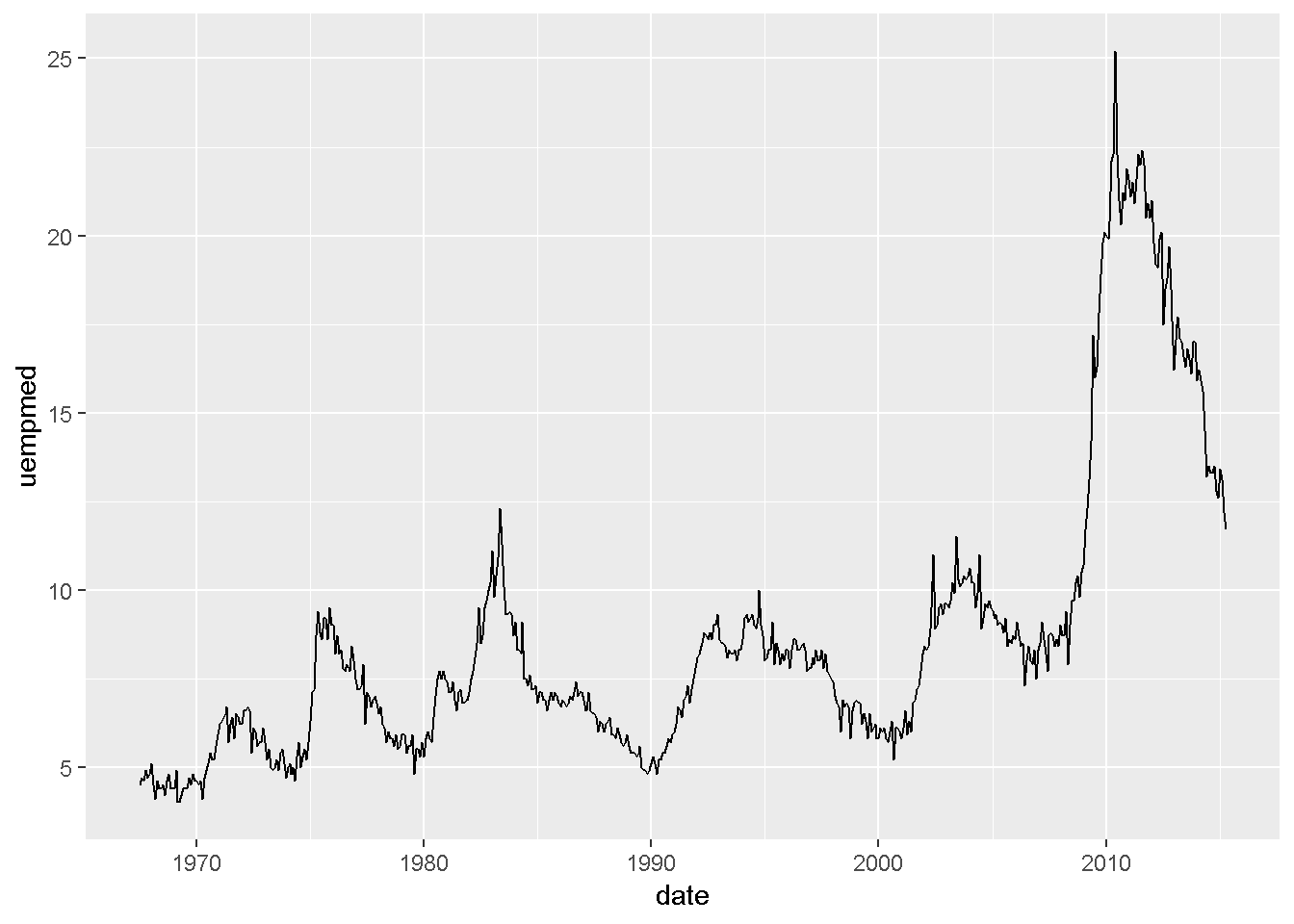``VI(g13b)   ``
``````## This is an untitled chart with no subtitle or caption.
## It has x-axis 'date' with labels 1970, 1980, 1990, 2000 and 2010.
## It has y-axis 'uempmed' with labels 5, 10, 15, 20 and 25.
## The chart is a set of 1 line.
## Line 1 connects 574 points.``````
``````# path plots
year <- function(x) as.POSIXlt(x)\$year + 1900
g14a = qplot(unemploy / pop, uempmed, data = economics, geom = c("point", "path"))
# summary(g14a)
g14a``````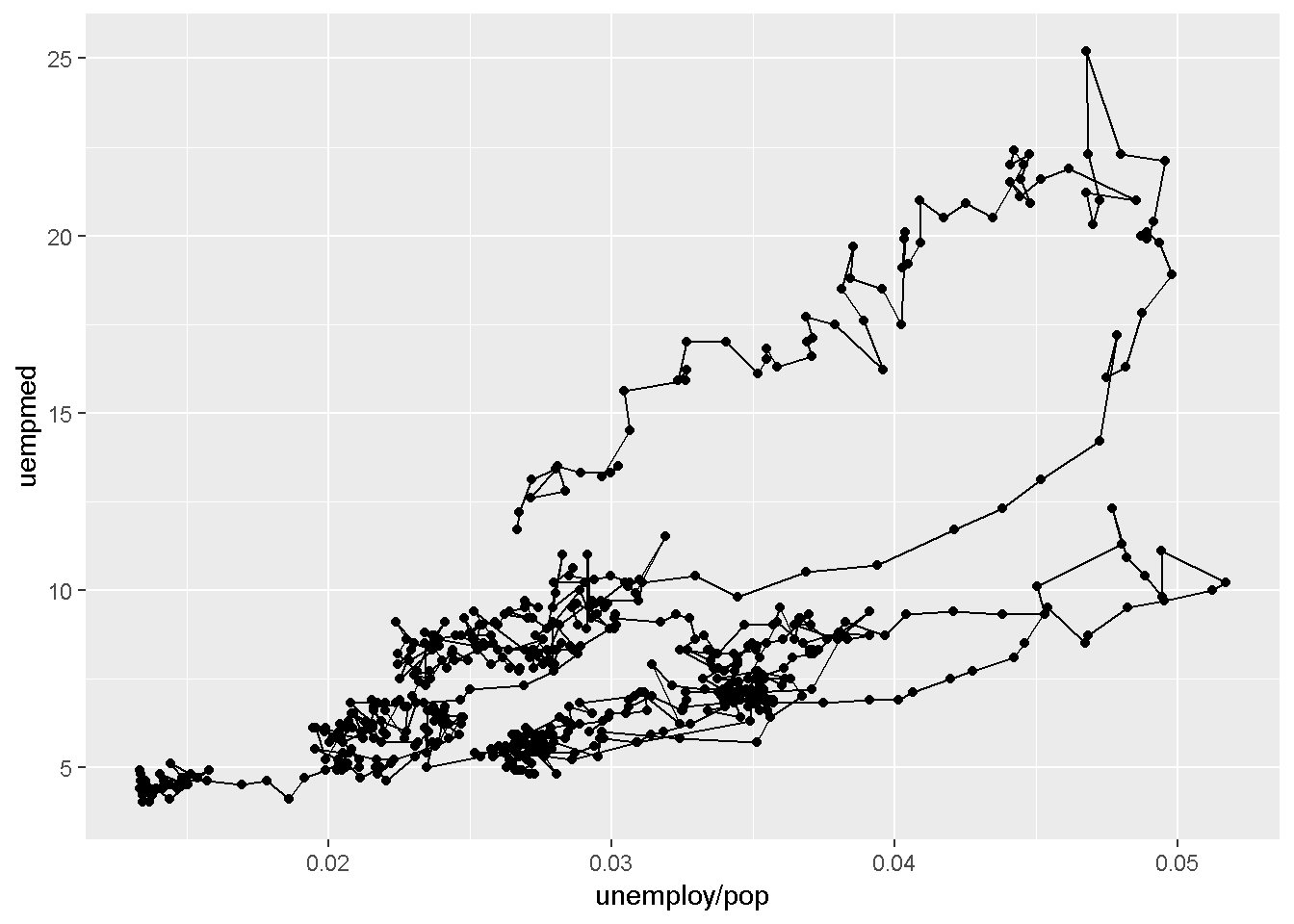``VI(g14a)   ``
``````## This is an untitled chart with no subtitle or caption.
## It has x-axis 'unemploy/pop' with labels 0.02, 0.03, 0.04 and 0.05.
## It has y-axis 'uempmed' with labels 5, 10, 15, 20 and 25.
## It has 2 layers.
## Layer 1 is a set of 574 points.
## Layer 2 is a type that VI isn't able to process.``````
``````#g14b = qplot(unemploy / pop, uempmed, data = economics, geom = "path", colour = year(date)) + scale_area()
#summary(g14b)
#VI(g14b)   ``````
``````# facets is the ggplot term for trellis' panels
g15a = qplot(carat, data = diamonds, facets = color ~ ., geom = "histogram", binwidth = 0.1, xlim = c(0, 3))
# summary(g15a)
g15a``````
``## Warning: Removed 32 rows containing non-finite values (stat_bin).``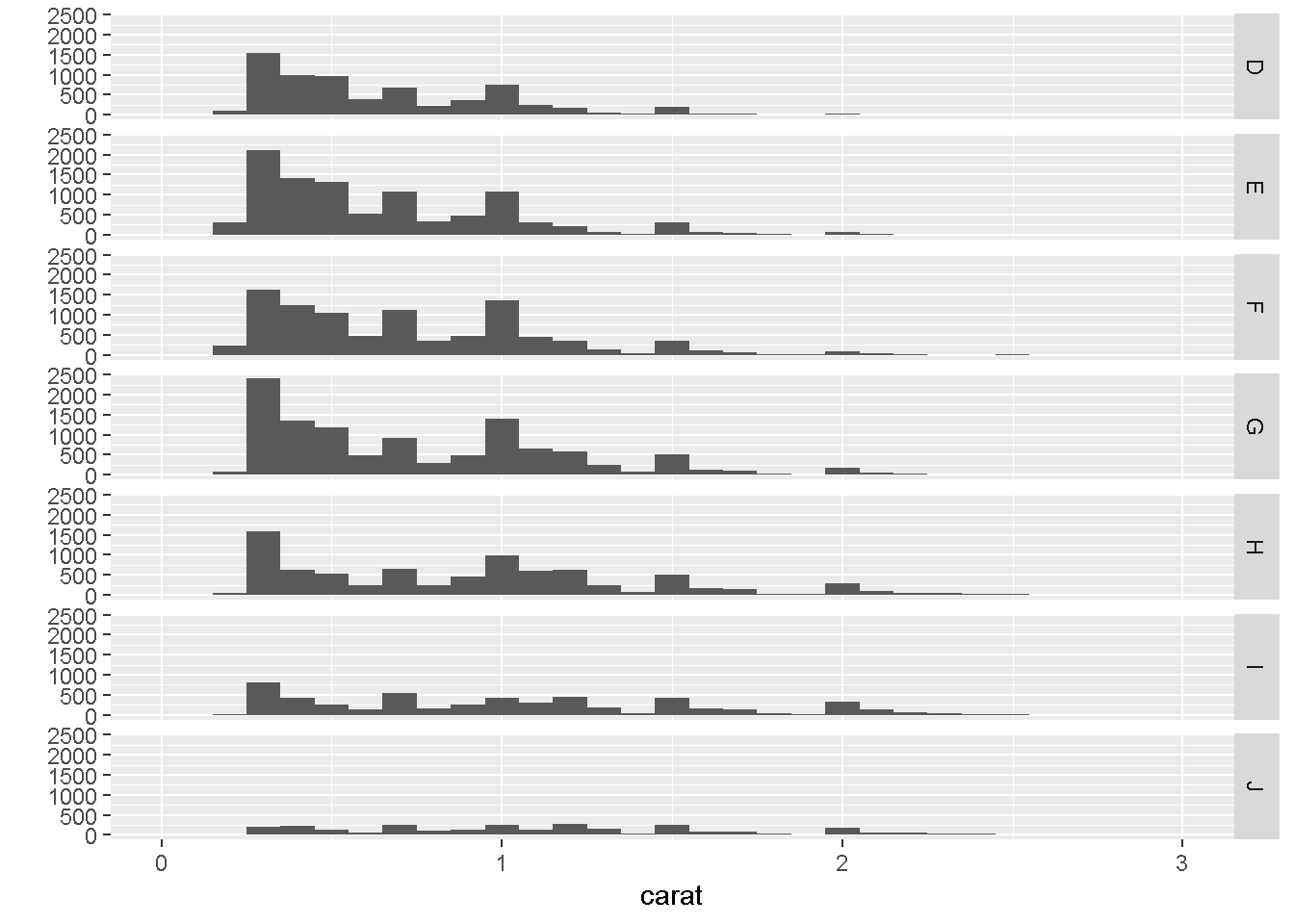``VI(g15a)   ``
``## Warning: Removed 32 rows containing non-finite values (stat_bin).``
``````## This is an untitled chart with no subtitle or caption.
## The chart is comprised of 7 panels containing sub-charts, arranged vertically.
## The panels represent different values of color.
## Each sub-chart has x-axis 'carat' with labels 0, 1, 2 and 3.
## Each sub-chart has y-axis '' with labels 0, 500, 1000, 1500, 2000 and 2500.
## Panel 1 represents data for color = D.
## Panel 1 is a bar chart containing 29 vertical bars.
## Panel 2 represents data for color = E.
## Panel 2 is a bar chart containing 29 vertical bars.
## Panel 3 represents data for color = F.
## Panel 3 is a bar chart containing 29 vertical bars.
## Panel 4 represents data for color = G.
## Panel 4 is a bar chart containing 29 vertical bars.
## Panel 5 represents data for color = H.
## Panel 5 is a bar chart containing 29 vertical bars.
## Panel 6 represents data for color = I.
## Panel 6 is a bar chart containing 29 vertical bars.
## Panel 7 represents data for color = J.
## Panel 7 is a bar chart containing 29 vertical bars.``````
``````g15b = qplot(carat, ..density.., data = diamonds, facets = color ~ ., geom = "histogram", binwidth = 0.1, xlim = c(0, 3))
# summary(g15b)
g15b``````
``## Warning: Removed 32 rows containing non-finite values (stat_bin).``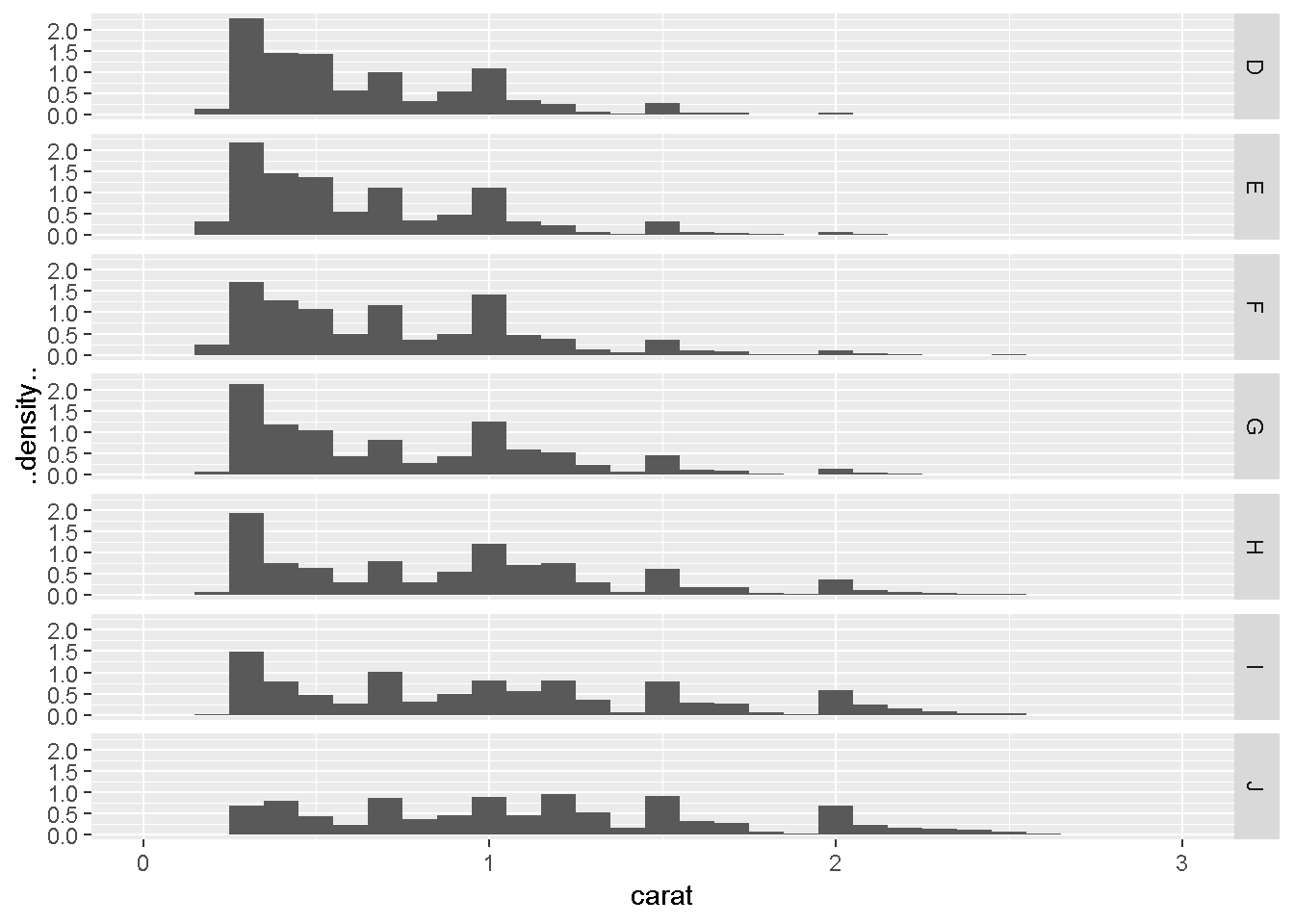``VI(g15b)   ``
``## Warning: Removed 32 rows containing non-finite values (stat_bin).``
``````## This is an untitled chart with no subtitle or caption.
## The chart is comprised of 7 panels containing sub-charts, arranged vertically.
## The panels represent different values of color.
## Each sub-chart has x-axis 'carat' with labels 0, 1, 2 and 3.
## Each sub-chart has y-axis '..density..' with labels 0.0, 0.5, 1.0, 1.5 and 2.0.
## Panel 1 represents data for color = D.
## Panel 1 is a bar chart containing 29 vertical bars.
## Panel 2 represents data for color = E.
## Panel 2 is a bar chart containing 29 vertical bars.
## Panel 3 represents data for color = F.
## Panel 3 is a bar chart containing 29 vertical bars.
## Panel 4 represents data for color = G.
## Panel 4 is a bar chart containing 29 vertical bars.
## Panel 5 represents data for color = H.
## Panel 5 is a bar chart containing 29 vertical bars.
## Panel 6 represents data for color = I.
## Panel 6 is a bar chart containing 29 vertical bars.
## Panel 7 represents data for color = J.
## Panel 7 is a bar chart containing 29 vertical bars.``````
``````# rescaling of the axes
g16 = qplot(carat, price, data = dsmall, log = "xy")
# summary(g16)
g16``````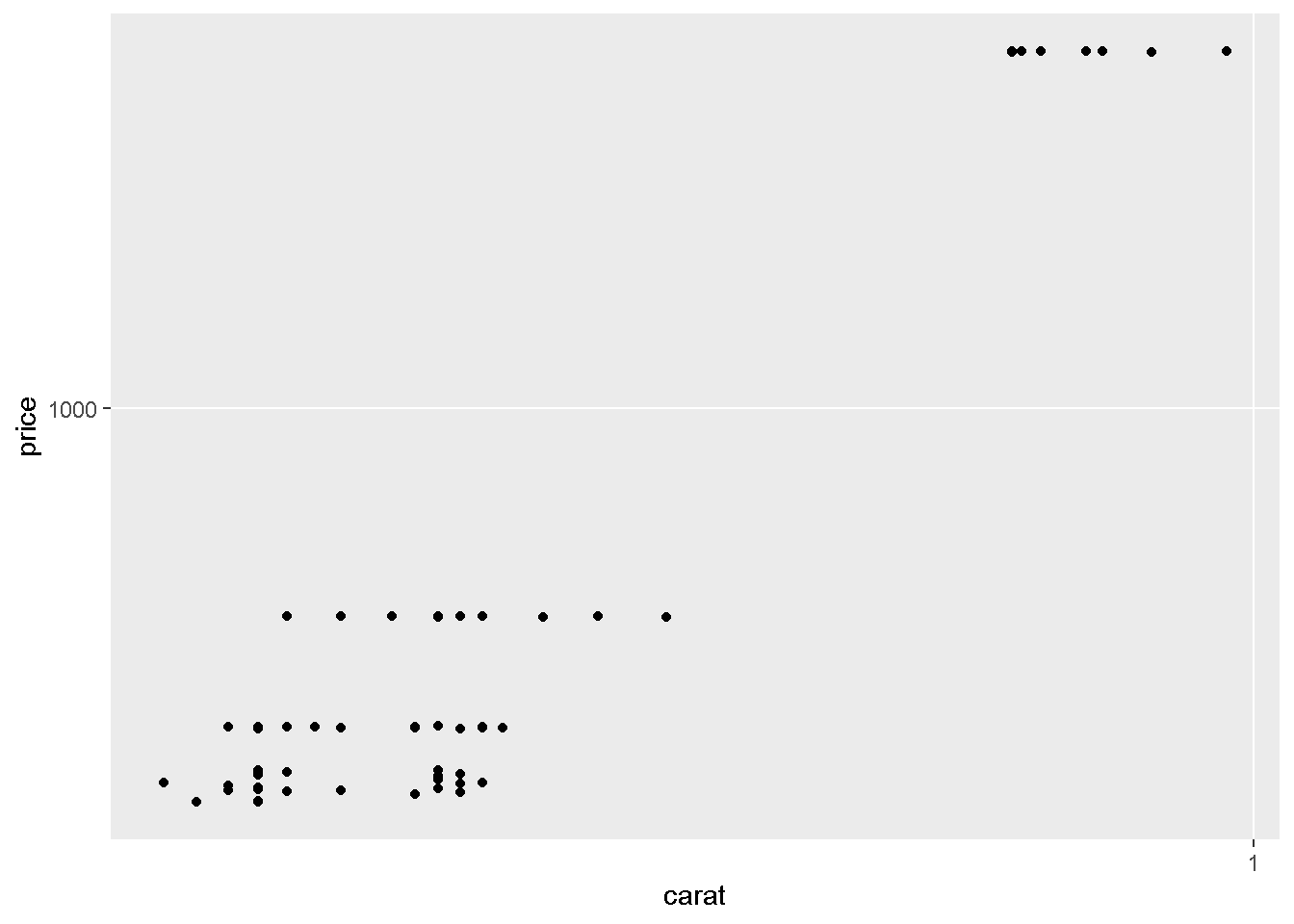``VI(g16)   ``
``````## This is an untitled chart with no subtitle or caption.
## It has x-axis 'carat' with labels 1.
## It has y-axis 'price' with labels 1000.
## The chart is a set of 100 points.``````
``````# Facets syntax without a "." before the "~" causes grief
g17 = qplot(displ, hwy, data=mpg, facets =~ year) + geom_smooth()
# summary(g17)
g17``````
``## `geom_smooth()` using method = 'loess' and formula 'y ~ x'``